# Equation of Straight Line JEE Notes | EduRev

## JEE : Equation of Straight Line JEE Notes | EduRev

The document Equation of Straight Line JEE Notes | EduRev is a part of the JEE Course Mathematics (Maths) Class 12.
All you need of JEE at this link: JEE

Straight Line

(i) Equation of a line through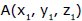and having direction cosines l , m , n are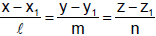and the lines through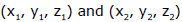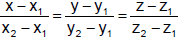(ii) Intersection of two planes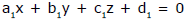and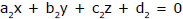together represent the unsymmetrical form of the straight line.
(iii) General equation of the plane containing the line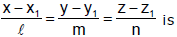A(x – x1) + B(y – y1) + c(z – z1) = 0where Al + bm + cn = 0.

(iv) Line of Greatest Slope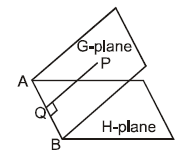AB is the line of intersection of G-plane and H is the horizontal plane. Line of greatest slope on a given plane, drawn through a given point on the plane, is the line through the point ‘P’ perpendicular to the line of intersection of the given plane with any horizontal plane.

Ex.15 Show that the distance of the point of intersection of the line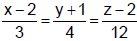and the plane x – y + z = 5 from the point (–1, –5, –10) is 13.

Sol. The equation of the given line are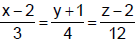= r (say).          ....(1)

The co-ordinates of any point on the line (1) are (3r + 2, 4r - 1, 12 r + 2). If this point lies on the plane x – y + z = 5, we have 3r + 2 – (4r – 1) + 12r + 2 = 5, or 11r = 0, or r = 0.
Putting this value of r, the co-ordinates of the point of intersection of the line (1) and the given plane are (2, –1, 2).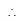The required distance = distance between the points (2, –1, 2) and (–1, –5, –10)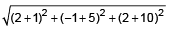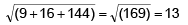Ex.16 Find the co-ordinates of the foot of the perpendicular drawn from the origin to the plane 3x + 4y – 6z + 1 = 0. Find also the co-ordinates of the point on the line which is at the same distance from the foot of the perpendicular as the origin is.

Sol. The equation of the plane is 3x + 4y – 6z + 1 = 0.  ....(1)
The direction ratios of the normal to the plane (1) are 3, 4, –6. Hence the line normal to the plane (1) has d.r.’s 3, 4, –6, so that the equations of the line through (0, 0, 0) and perpendicular to the plane (1) are x/3 = y/4 = z/–6 = r ....(2)

The co-ordinates of any point P on (2) are (3r, 4r, – 6r)   ....(3)

If this point lies on the plane (1), then 3(3r) + r(4r) – 6(–6r) + 1 = 0, or r = –1/61.

Putting the value of r in (3), the co-ordinates of the foot of the perpendicular P are (–3/61, –4/61, 6/61).
Now let Q be the point on the line which is at the same distance from the foot of the perpendicular as the origin. Let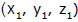be the co-ordinates of the point Q. Clearly P is the middle point of OQ.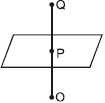Hence we have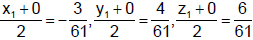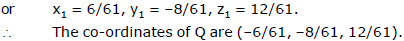Ex.17 Find in symmetrical form the equations of the line 3x + 2y – z – 4 = 0 & 4x + y – 2z + 3 = 0 and find its direction cosines.

Sol. The equations of the given line in general form are 3x + 2y – z – 4 = 0 & 4x + y – 2z + 3 = 0 ..(1)

Let l, m, n be the d.c.’s of the line. Since the line is common to both the planes, it is perpendicular to the normals to both the planes. Hence we have 3l + 2m – n = 0,            4l + m – 2n = 0.

Solving these, we get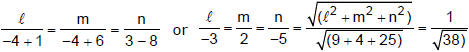∴ the d.c.’s of the line are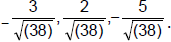Now to find the co-ordinates of a point on the line given by (1), let us find the point where it meets the plane z = 0. Putting z = 0 i the equations given by (1), we have 3x + 2y – 4 = 0, 4x + y + 3 = 0.

Solving these, we get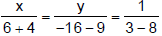, or x = –2, y = 5.
Therefore the equation of the given line in symmetrical form is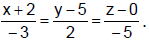Ex.18 Find the equation of the plane through the line 3x – 4y + 5z = 10, 2x + 2y – 3z = 4 and parallel to the line x = 2y = 3z.

Sol. The equation of the given line are 3x – 4y + 5z = 10, 2x + 2y – 3z = 4    ...(1)
The equation of any plane through the line (1) is (3x – 4y + 5z – 10) + λ (2x + 2y – 3z – 4) = 0
or (3 + 2λ)x + (–4 +2λ) y + (5 – 3λ) z – 10 – 4λ = 0.   ...(2)
The plane (1) will be parallel to the line x = 2y = 3z  i.e.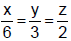if
(3 + 2λ) . 6 + (–4 + 2λ). 3 + (5 – 3λ).2 = 0  or

λ(12 + 6 – 6) + 18 – 12 + 10 = 0 or λ = –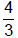.

Putting this value of λ in (2), the required equation of the plane is given by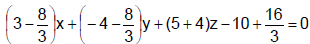or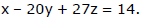Ex.19 Find the equation of a plane passing through the line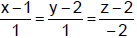and making an angle of 30° with the plane x + y + z = 5.
Sol. The equation of the required plane is (x – y + 1) + λ(2y + z – 6) = 0 ⇒ x + (2λ – 1) y + λz + 1 – 6λ = 0

Since it makes an angle of 30° with x +y + z = 5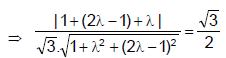⇒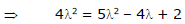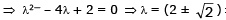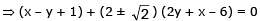are two required planes.

Ex.20 Prove that the lines 3x + 2y + z – 5 = 0 = x + y – 2z – 3 and 2x – y – z = 0 = 7x + 10y – 8z – 15 are perpendicular.

Sol. Let l1, m1, n1 be the d.c.s of the first line. Then 3l1 + 2m1 + n= 0, l1 + m1 - 2n1 = 0. Solving, we get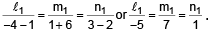Again let l2, m2,n2 be the d.c.'s of the second line, then 2l2 - m2 - n2 = 0, 7l2 + 10m2 - 8n2 = 0.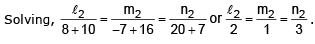Solving,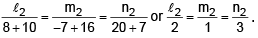Hence the d.c.’s of the two given lines are proportional to –5, 7, 1 and 2, 1, 3. We have

–5.2 + 7.1 + 1.3 = 0 ∴the given lines are perpendicular.

Ex.21 Find the equation of the plane which contains the two parallel lines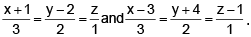Sol. The equation of the two parallel lines are

(x + 1)/3 = (y – 2)/2 = (z – 0)/1 ....(1) and (x – 3)/3 = (y + 4)/2 = (z – 1)/1. ....(2)

The equation of any plane through the line (1) is

a(x + 1) + b (y – 2) + cz = 0, ....(3)

where  3a + 2b + c = 0. ....(4)

The line (2) will also lie on the plane (3) if the point (3, –4, 1) lying on the line (2) also lies on the plane (3), and for this we have a (3 + 1) + b (–4 – 2) + c. 1 = 0 or 4a – 6b + c = 0. ....(5)

Solving (4) and (5), we get a/8 = b/1 = c/-24

Putting these proportionate values of a, b, c in (3), the required equation of the plane is

8(x + 1) + 1.(y – 2) – 26z = 0, or 8x + y – 26 + 6 = 0.

Ex.22 Find the distance of the point P(3, 8, 2) from the line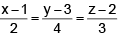measured parallel to the plane 3x + 2y – 2z + 17 = 0.

Sol .The equation of the given line are (x – 1)/2 = (y – 3)/4 = (z – 2)/3 = r, (say). ...(1)

Any point Q on the line (1) is (2r + 1, 4r + 3, 3r + 2)

Now P is the point (3, 8, 2) and hence d.r.’s of PQ are

2r + 1 – 3, 4r + 3 – 8, 3r + 2 – 2 i.e. 2r – 2, 4r – 5, 3r.

It is required to find the distance PQ measured parallel to the plane 3x + 2y – 2z + 17 = 0   ...(2)

Now PQ is parallel to the plane (2) and hence PQ will be perpendicular to the normal to the plane (2).

Hence we have (2r – 2) (3) + (4r – 5) (2) + (2r) (–2) = 0 or 8r – 16 = 0, or r = 2.

Putting the value of r, the point Q is (5, 11, 8) =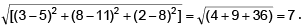Ex.23 Find the projection of the line 3x – y + 2z = 1, x + 2y – z = 2 on the plane 3x + 2y + z = 0.

Sol. The equations of the given line are 3x – y + 2z = 1, x + 2y – z = 2. ...(1)

The equation of the given plane is 3x + 2y + z = 0. ...(2)

The equation of any plane through the line (1) is (3x – y + 2z – 1) + l(x + 2y - z - 2) = 0

or (3 + l) x + (-1 + 2l) y + (2 - l) z - 1 - 2l = 0 ....(3)

The plane (3) will be perpendicular to the plane (2), if 3(3 + l)+ 2(-1 + 2l) + 1 (2 - l) = 0 or l = -3/3.

Putting this value of l in (3), the equation of the plane through the line (1) and perpendicular to the plane (2) is given by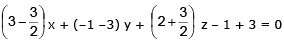or 3x – 8y + 7z + 4 = 0. ....(4)

The projection of the given line (1) on the given plane (2), is given by the equations (2) and (4) together.

Ex.25 Find the foot and hence the length of the perpendicular from the point (5, 7, 3) to the line (x – 15)/3 = (y – 29)/8 = (z – 5)/(–5). Find the equations of the perpendicular. Also find the equation of the plane in which the perpendicular and the given straight line lie.

Sol. Let the given point (5, 7, 3) be P.

The equations of the given line are (x – 15)/3 = (y – 29)/8 = (z – 5)/(–5)= r (say). ...(1)

Let N be the foot of the perpendicular from the point P to the line (1). The co-ordinates of N may be taken as (3r + 15, 8r + 29, – 5r + 5). ...(2)

the direction ratios of the perpendicular PN are

3r + 15 – 5, 8r + 29 – 7, – 5r + 5 – 3,  i.e. are 3r + 10, 8r + 22, –5r + 2. ...(3)

Since the line (1) and the line PN are perpendicular to each other, therefore

3 (3r + 10) + 8 (8r + 22) – 5(–5r + 2) = 0 or 98r + 196 = 0 or r = –2

Putting this value of r in (2) and (3), the foot of the perpendicular N is (9, 13, 15) and the direction ratios of the perpendicular PN are 4, 6, 12 or 2, 3, 6.

the equations of the perpendicular PN are (x – 5)/2 = (y – 7)/3 = (z – 3)/6. ...(4)

Length of the perpendicular PN

= the distance between P(5, 7, 3) and N(9, 13, 15) =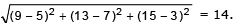Lastly the equation of the plane containing the given line (1) and the perpendicular (4) is given by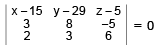or (x – 15) (48  + 15) – (y – 29) (18 + 10) + (z – 5) (9 – 16) = 0 or  9x – 4y – z =– 14 = 0.

Ex.26 Show that the planes 2x – 3y – 7z = 0, 3x – 14y – 13z = 0, 8x – 31y – 33z = 0 pass through the one line find its equations.

Sol .The rectangular array of coefficient is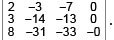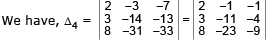(by C2 + C1, C2 + 3C1)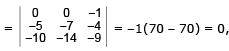(by C1 + 2C2, C– C2)

since Δ= 0, therefore, the three planes either intersect in a line or form a triangular prism.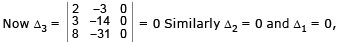Hence the three planes intersect in a common line.

Clearly the three planes pass through (0, 0, 0) and hence the common line of intersection will pass through (0, 0, 0). The equations of the common line are given by any of the two given planes. Therefore the equations of the common line are given by 2x – 3y – 7z = 0  and  3x – 14y – 13z = 0.

∴ the symmetric form of the line is given by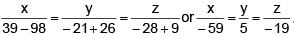Ex.27 For what values of k do the planes  x – y + z + 1 = 0, kx + 3y + 2z – 3 = 0, 3x + ky + z – 2 = 0 (i) intersect in a point ; (ii) intersect in a line ; (iii) form a triangular prism ?

Sol. The rectangular array of coefficients is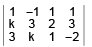Now we calculate the following determinants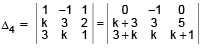(adding 2nd column to 1st and 3rd)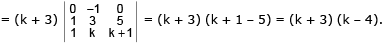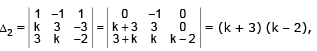(adding 2nd column to 1st and 3rd)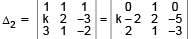(adding (–1) times 2nd column to 1st and 3rd)

= –{(k – 2) (–3) + 10} = 3k – 16, and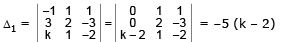(adding 3rd column to 1st)

(i) The given planes will intersect in a point if Δ4 ≠ 0 and so we must have k ≠ -3 and k ≠ 4. Thus the given planes will intersect in a point for all real values of k other than -3 and 4.

(ii) If k = –3, we have Δ4 = 0, Δ3 = 0 but D2 ≠ 0. Hence the given planes will form a triangular prism if k = -3.

(iii) If k = 4, we have Δ4 = 0 but Δ3 ≠ 0. Hence the given planes will form a triangular prism if k = 4.
We observe that  for no value of k the given planes will have a common line of intersection.

Ex.28 Find the equation of the line passing through (1, 1, 1) and perpendicular to the line of intersection of the planes x + 2y – 4z = 0 and 2x – y + 2z = 0.

Sol. Equation of the plane through the lines x + 2y – 4z = 0 and 2x – y + 2z = 0 is

x + 2y – 4z + l (2x - y + 2z) = 0 ...(1)

If (1, 1, 1) lies on this plane, then –1 + 3l = 0

⇒ l = 1/3 , so that the plane becomes 3x + 6y – 12z + 2x – y + 2z = 0 ⇒ x + y – 2z = 0 ....(2)

Also (1) will be perpendicular to (2) if 1 + 2l + 2 - l - 2(-4 + 2l) = ⇒ l = 11/3

Equation of plane perpendicular to (2) is 5x – y + 2z = 0. ...(3)

Therefore the equation of line through (1, 1, 1) and perpendicular to the given line is parallel to the normal to the plane (3). Hence the required line is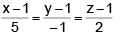Alternate :

Solving the equation of planes x + 2y – 4z = 0 and 2x – y + 2z = 0, we get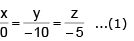Any point P on the line (1) can be written as (0, –10l, -5l).

Direction ratios of the line joining P and Q(1, 1, 1) is (1, 1, + 10l, 1 + 5l).

Line PQ is perpendicular to line (1) ⇒ 0(1) – 10(1 + 10l) - 5(1 + 5l) = 0

⇒  0 - 10 - 100l - 5 - 25x = 0 or 125l + 15 = 0  ⇒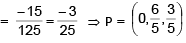Direction ratios of PQ =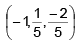Hence equations of lien are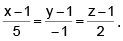Ex.29 Find the shortest distance (S.D.) between the lines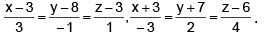Find also its equations and the points in which it meets the given lines.

Sol. The equations of the given lines are (x – 3)/3 = (y – 8)/–1 = (z – 3)/1 = r1 (say) ...(1)

and (x + 3)/(–3) = (y + 7)/2 = (z – 6)/4 = r2 (say) ..(2)

Any point on line (1) is (3r1 + 3, –r1 + 8, r1 + 3), say P. ...(3)

any point on line (2) is (–3r2 – 3, 2r2 – 7, 4r2 + 6), say Q. ..(4)

The d.r.’s of the line PQ are (–3r– 3) – (3r1 + 3), (2r2 – 7) – (–r1 + 8), (4r2 + 6) – (r1 + 3)

or –3r2 – 3r1– 6, 2r2 + r1 – 15, 4r2 – r1 + 3. ..(5)

Let the line PQ be the lines of S.D., so that PQ is perpendicular to both the given lines (1) and (2), and so we have

3 (–3r2 – 3r1 – 6) – 1 (2r2 + r1 – 15) + 1. (4r2 – r1 + 3) = 0

and –3(–3r2 – 3r1 – 6) + 2. (2R+ r1 – 15) + 4 (4r2 – r1 + 3) = 0

or –7r2 – 11r1 = 0 and 11r2 + 7r1 = 0

Solving these equations, we get r1 = r2 = 0.

Substituting the values of r1 and r2 in (3), (4) and (5), we have P(3, 8, 3), Q(–3, –7, 6) And the d.r.’s of PQ (the line of S.D.) are –6, –15, 3 or –2, –5, 1.

The length of S.D. = the distance between the points P and Q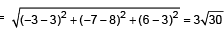Now the line PQ of shortest distance is the line passing through P(3, 8, 3) and having d.r.’s –2, –5, 1 and hence its equations are given by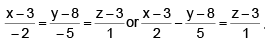Offer running on EduRev: Apply code STAYHOME200 to get INR 200 off on our premium plan EduRev Infinity!

## Mathematics (Maths) Class 12

209 videos|222 docs|124 tests

,

,

,

,

,

,

,

,

,

,

,

,

,

,

,

,

,

,

,

,

,

;